Thermodynamics and Propulsion

# 19.2 Kirchhoff's Law and Real Bodies''

Real bodies radiate less effectively than black bodies. The measurement of this is the emittance,, defined bywhere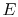is radiation from the real body at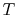, and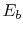is radiation from a black body at.

Values of emittance vary greatly for different materials. They are near unity for rough surfaces such as ceramics or oxidized metals, and roughly 0.02 for polished metals or silvered reflectors. A table of emittances for different substances is given at the end of this section as Table 19.1, taken from the book by Lienhard.

The level of the emittance can be related to the absorptance using the following arguments. Suppose we have a small non-black body in the cavity. The power absorbed per unit area is equal to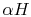. The power emitted is equal to. An energy balance gives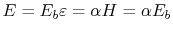. Thus(19..1)

Equation (19.1), the relation, is known as Kirchhoff's Law. It implies that good radiators are good absorbers. It was derived for the case whenand is not strictly true for all circumstances when the temperature of the body and the cavity are different, but it is true if,, so the absorptance and emittance are not functions of. This situation describes a gray body.'' Also, since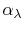,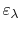are properties of the surface,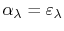.

UnifiedTP